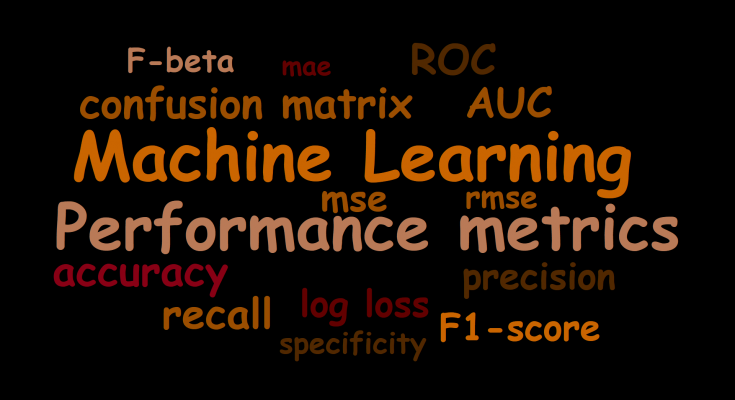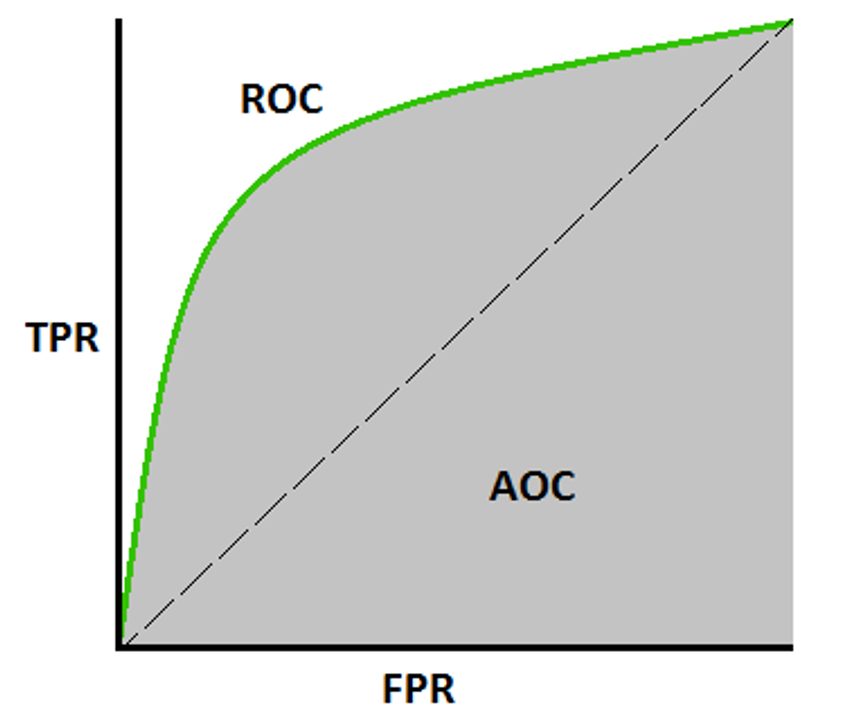# Performance Metrics in Machine Learning Classification ModelToday we are going to talk about 5 of the most widely used Evaluation Metrics of Classification Model. Before going into the details of performance metrics, let’s answer a few points:

##### Why do we need Evaluation Metrics?

Being Humans we want to know the efficiency or the performance of any machine or software we come across. For example, if we consider a car we want to know the Mileage, or if we there is a certain algorithm we want to know about the Time and Space Complexity, similarly there must be some or the other way we can measure the efficiency or performance of our Machine Learning Models as well.

That being said, let’s look at some of the metrics for our Classification Models. Here, there are separate metrics for Regression and Classification models. As Regression gives us continuous values as output and Classification gives us discrete values as output, we will focus on Classification Metrics.

#### ACCURACY

The most commonly and widely used metric, for any model, is accuracy, it basically does what It says, calculates what is the prediction accuracy of our model. Accuracy in machine learning is a metric that quantifies the correctness of a model’s predictions. It measures the proportion of correct predictions made by the model out of all the predictions it has made. The formulation is given below:

As we can see, it basically tells us among all the points how many of them are correctly predicted.

• 1. Easy to use Metric.
• 2. Highly Interpretable.
• 3. If data points are balanced it gives proper effectiveness of the model.

• 1. Not recommended for Imbalanced data, as results can be misleading. Let me give you an example. Let’s say we have 100 data points among which 95 points are negative and 5 points are positive. If I have a dumb model, which only predicts negative results then at the end of training I will have a model that will only predict negative. But still, be 95% accurate based on the above formula. Hence not recommended for imbalanced data.
• 2. We don’t understand where our model is making mistakes.

#### CONFUSION METRICS

A confusion matrix in AI is a tabular representation used to assess the performance of a classification model. It compares the predicted classifications made by the model against the actual known classifications in a dataset. The matrix provides a breakdown of the counts of true positives, true negatives, false positives, and false negatives. These values are then utilized to calculate various metrics that offer insights into the model’s accuracy and effectiveness in making predictions.

The confusion matrix is particularly useful because it provides a more detailed understanding of how well a classification model is performing across different classes. From the confusion matrix, various metrics like accuracy, precision, recall, F1-score, and more can be computed. These metrics help evaluate the model’s strengths and weaknesses, especially in scenarios where certain classes might be more critical or more challenging to predict accurately. Overall, the confusion matrix aids in refining models, fine-tuning algorithms, and making informed decisions about the model’s deployment and optimization.

Let’s have a look at the diagram to have a better understanding of it:

##### What does these notation means?

Imagine I have a binary classification problem with classes as positive and negative labels, now, If my actual point is Positive and my Model predicted point is also positive then I get a True Positive, here “True” means correctly classified and “Positive” is the predicted class by the model, Similarly If I have actual class as Negative and I predicted it as Positive, i.e. an incorrect predicted, then I get False Positive, “False” means Incorrect prediction, and “Positive” is the predicted class by the model.

We always want diagonal elements to have high values. As they are correct predictions, i.e. TP & TN.

• 1. It specifies a model is confused between which class labels.
• 2. You get the types of errors made by the model, especially Type I or Type II.
• 3. Better than accuracy as it shows the incorrect predictions as well, you understand in-depth the errors made by the model, and rectify the areas where it is going incorrect.

• 1. Not very much well suited for Multi-class.

#### PRECISION & RECALL

Precision is a metric used in machine learning to evaluate the accuracy of positive predictions made by a model. It assesses how many of the instances that the model classified as positive are actually true positives. In other words, precision measures the proportion of correctly identified positive cases out of all the instances that the model predicted as positive. Precision is the measure which states, among all the predicted positive classes, how many are positive, the formula is given below:

Recall, also known as sensitivity or true positive rate, is a metric used in machine learning to assess a model’s ability to identify all positive instances in a dataset. It measures the proportion of actual positive cases that the model correctly predicts as positive. In other words, recall quantifies how well the model avoids missing true positive cases. Recall is the measure that states, among all the Positive classes, how many are predicted correctly. The formula is given below:

A high recall indicates that the model is proficient at capturing most positive cases, even if it means making more false positive errors. In scenarios where missing positive instances is more critical than incorrectly classifying negatives, prioritizing recall might be essential. Precision and recall are often considered to evaluate the trade-off between accurate positive predictions and minimizing missed positive cases.

We often seek to get high precision and recall. If both are high means, our model is sensible. Here, we also take into consideration the incorrect points. Hence we are aware where our model is making mistakes, and Minority class is also taken into consideration.

• 1. It tells us about the efficiency of the model
• 2. Also shows us how much of the data is biased toward one class.
• 3. Helps us understand whether our model is performing well in an imbalanced dataset for the minority class.

• 1. Recall deals with true positives and false negatives, and precision deals with true positives and false positives. It doesn’t deal with all the cells of the confusion matrix. True negatives are never taken into account.
• 2. Hence, precision and recall should only be used in situations where the correct identification of the negative class does not play a role.
• 3. Focuses only on Positive class.
• 4. Best suited for Binary Classification.

#### F1-SCORE

F1 score is the harmonic mean of the precision and recall, where an F1 score reaches its best value at 1 (perfect precision and recall). The F1 score is also known as the Sorensen–Dice or Dice similarity coefficients (DSC).

It leverages both the advantages of Precision and Recall. An Ideal model will have precision and recall as 1 hence F1 score will also be 1.

• 1. It is the same as Precision and Recall.

#### AU-ROC

AUC ROC, which stands for Area Under the Receiver Operating Characteristic Curve, is a widely used evaluation measure in machine learning for assessing binary classification models. It gauges how well a model can distinguish between positive and negative classes by examining its performance across different threshold settings.

The ROC curve charts the true positive rate (sensitivity) against the false positive rate (1 – specificity) at various classification thresholds. The AUC ROC value is then computed based on the area beneath this curve. Ranging between 0 and 1, a higher AUC ROC value signifies better model performance.

In simpler words, AUC ROC reflects the model’s ability to correctly identify positive and negative instances, regardless of the specific threshold chosen for classification. A higher AUC ROC value suggests the model is adept at differentiation, while a value closer to 0.5 indicates performance similar to random guessing. This metric proves valuable when dealing with imbalanced datasets or scenarios where the costs of false positives and false negatives differ.

As AU-ROC is a graph, it has its own X-axis and Y-axis, whereas X-axis is FPR and Y-axis is TPR
TPR = True Positive / (True Positive + False Negative)
FPR = False Positive / (False Positive + True Negative)

ROC curve plots are basically TPR vs. FPR calculated at different classification thresholds. Lowering the classification threshold classifies more items as positive, thus increasing both False Positives and True Positives i.e., basically correct predictions.

All the values are sorted and plotted in a graph, and the area under the ROC curve is the model’s actual performance at different thresholds.• 1. A simple graphical representation of the diagnostic accuracy of a test: the closer the curve’s apex toward the upper left corner, the greater the discriminatory ability of the test.
• 2. Also, it allows a more complex (and more exact) measure of the accuracy of a test, which is the AUC.
• 3. The AUC, in turn, can be used as a simple numeric rating of diagnostic test accuracy, which simplifies comparison between diagnostic tests.

• 1. Actual decision thresholds are usually not displayed in the plot.
• 2. As the sample size decreases, the plot becomes more jagged.
• 3. Not easily interpretable from a business perspective.

#### SUMMARY

Classification performance metrics in machine learning provide a comprehensive evaluation of model effectiveness. Accuracy quantifies overall correctness, while precision gauges the proportion of correctly predicted positives, recall assesses the ability to identify all actual positives, and F1-score combines precision and recall for balanced performance assessment. Area Under the ROC Curve (AUC ROC) measures a model’s capability to distinguish classes at varying thresholds. These metrics aid in understanding trade-offs between different types of errors, ensuring informed decision-making based on the specific context and requirements of the classification task.

##### Recent Posts🚀 I'm Diwas Pandey, a Computer Engineer with an unyielding passion for Artificial Intelligence, currently pursuing a Master's in Computer Science at Washington State University, USA. As a dedicated blogger at AIHUBPROJECTS.COM, I share insights into the cutting-edge developments in AI, and as a Freelancer, I leverage my technical expertise to craft innovative solutions. Join me in bridging the gap between technology and healthcare as we shape a brighter future together! 🌍🤖🔬

View all posts by Diwas →

### 5 Comments on “Performance Metrics in Machine Learning Classification Model”

1.Chainey82256 says:

I find It really useful & it helped me out a lot

2.zortilonrel says:

3.renato says: